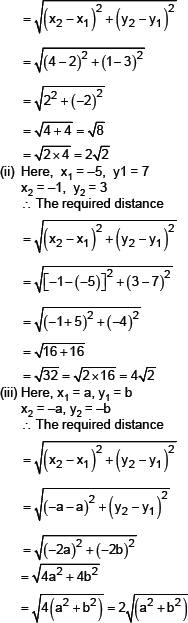# S.K.NEET

## Square and Square Roots

(i)   1225
(ii)   2601

#### Formulae used :

Distance Formula to find distance between two points (x1,y1) and (x2,y2) is :             D = √[(x2 – x1)2 + (y2 – y1)2 ]

#### Solution :

Sol. (i) Here x1 = 2, y1 = 3, x2 = 4 and y2 = 1
∴ The required distance(i)   1225
(ii)   2601

(i)   1225
(ii)   2601

(i)   1225
(ii)   2601

(i)   1225
(ii)   2601

(i)   1225
(ii)   2601

(i)   1225
(ii)   2601

(i)   1225
(ii)   2601

(i)   1225
(ii)   2601

(i)   1225
(ii)   2601

(i)   1225
(ii)   2601

(i)   1225
(ii)   2601

(i)   1225
(ii)   2601

(i)   1225
(ii)   2601